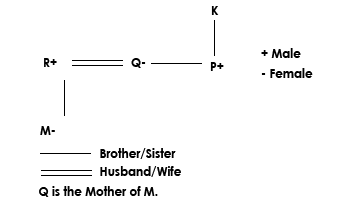# SML-ISUZU Reasoning Question

DIRECTIONS for question 1 to 4: Study the following information and answer the following question.
Seven people- J, K, L, M, N, O and P are sitting around a circular table facing the centre with equal distance between them but not necessarily in the same order. O sits second to the right of L. Only three people sit between O and M. P sits second to the left of J. J is not an immediate neighbor of O. Only one person sits between P and K.
1. Who sits fourth to the right of K?
1. O
2. L
3. N
4. J
5. Other than those given as options
J sits fourth to the right of K.
2. If in the give arrangement all the people are made to sit in alphabetical order in clockwise direction starting from J, then the positions of how many will remain unchanged (excluding J}?
1. Three
2. More than Three
3. One
4. Two
5. None
If in the give arrangement all the people are made to sit in alphabetical order in clockwise direction starting from J, then position of all the persons will change (except J). Thus, E is the correct option.
1. P is the son of K. Q is the only sibling of P. R is the son-in-law of K. M is the daughter of R. How is Q related to M?
1. Aunt
2. Brother
3. Father
4. Uncle
5. Mother2. In a class of 12 students, only three students rank higher than Sachin and only two students rank lower than Mukesh. How many students have their marks exactly between Sachin and Mukesh?
1. Cannot be determined
2. Three
3. Two
4. Five
5. Four
In a class of 12 students, only three students rank higher than Sachin It means after Sachin there are exactly 12-4= 8 students and also given that only two students rank lower than Mukesh. Therefore, students between sachin and Mukesh are = 8-3= 5 students have their marks exactly between them.
DIRECTIONS for question 5 to 7: In this question, relationship between different elements is shown in the statement. The statements are followed by two conclusions. Study the conclusions based on the given statement and select the appropriate answer.
3. Statement: D<I = A<R = E>S
Conclusion I: E > D
Conclusion II: I > S
1. Neither conclusion I nor II is true
2. Only conclusion I is true
3. Both conclusion I and II are true
4. Either conclusion I or II is true
5. Only conclusion II is true
D < I = A < R = E > S
Conclusion I: E > D, is true.
Conclusion II: I > S, is false.
Only Conclusion I is true.
4. Statement: A>N<G = E<L>S
Conclusion I: A>S
Conclusion II: L > N
1. Neither conclusion I nor II is true
2. Only conclusion I is true
3. Both conclusion I and II are true
4. Either conclusion I or II is true
5. Only conclusion II is true
A > N < G = E < L > S
Conclusion I: A > S, is false.
Conclusion II: L > N, is true.
Only Conclusion II is true.
5. Statement: E<X<C>L<U>D
Conclusion I: X>U
Conclusion II: E < D
1. Neither conclusion I nor II is true
2. Only conclusion I is true
3. Both conclusion I and II are true
4. Either conclusion I or II is true
5. Only conclusion II is true
E < X < C > L < U >D
Conclusion I: X > U, is False.
Conclusion II: E < D, is also False.
Neither conclusion I nor II is true
DIRECTIONS for question 8 to 10: The question is based on the five three-digit numbers given below:
568 378 654 496 476
6. What will be the resultant if third digit of the highest number is subtracted from the second digit of the smallest number?
1. 3
2. 5
3. 4
4. 2
5. 1
568 378 654 496 476
According to question, highest number of the series is 654. Smallest number of the series is 378. Third digit of the highest number which is 4, is subtracted from the second digit of the smallest number which is 7 then result 7-4=3.
7. If in all the numbers the position of first digit is interchanged with the third digit within the number, how many numbers thus formed will be divisible by 5?
1. Two
2. One
3. Three
4. More than Three
5. None